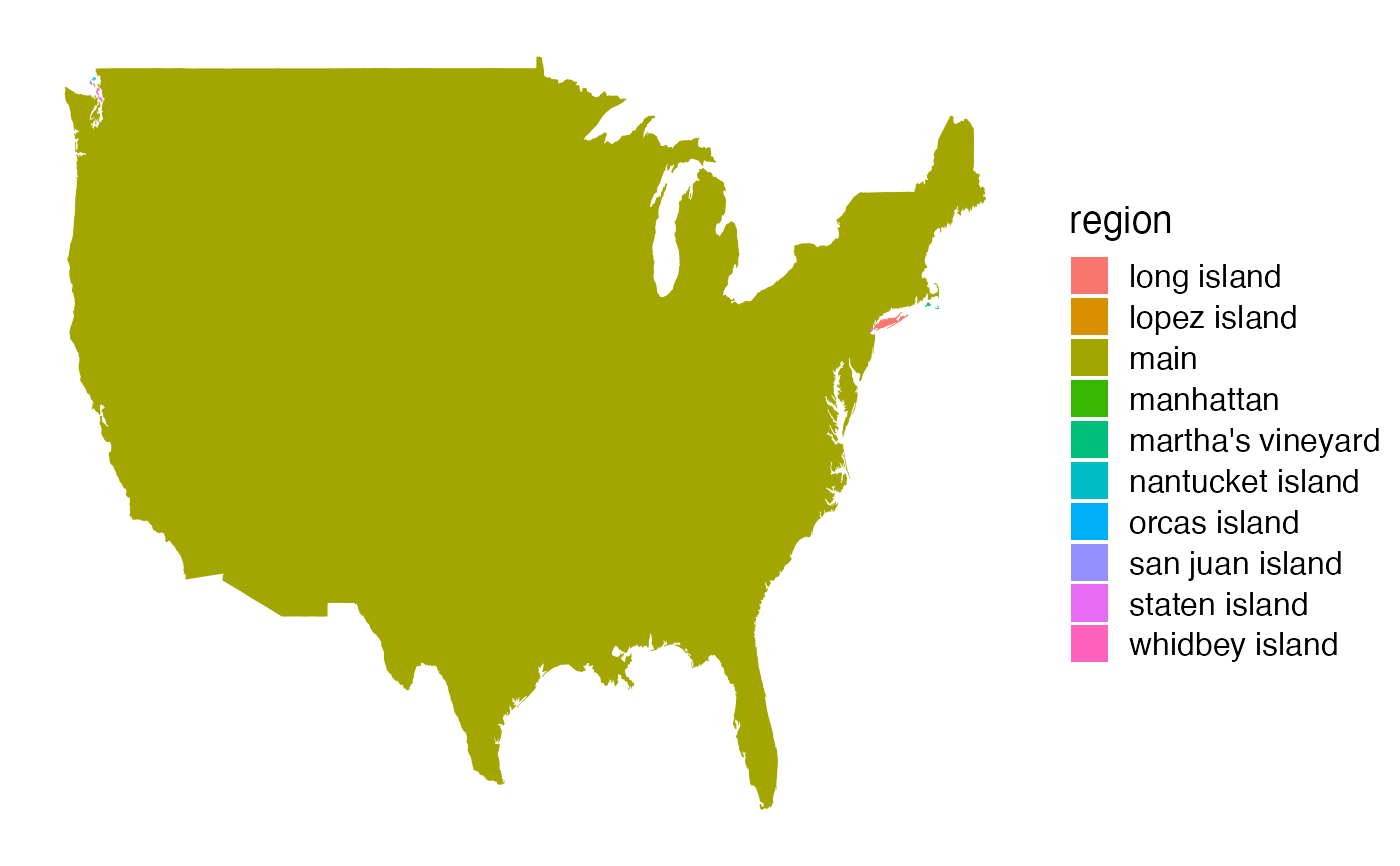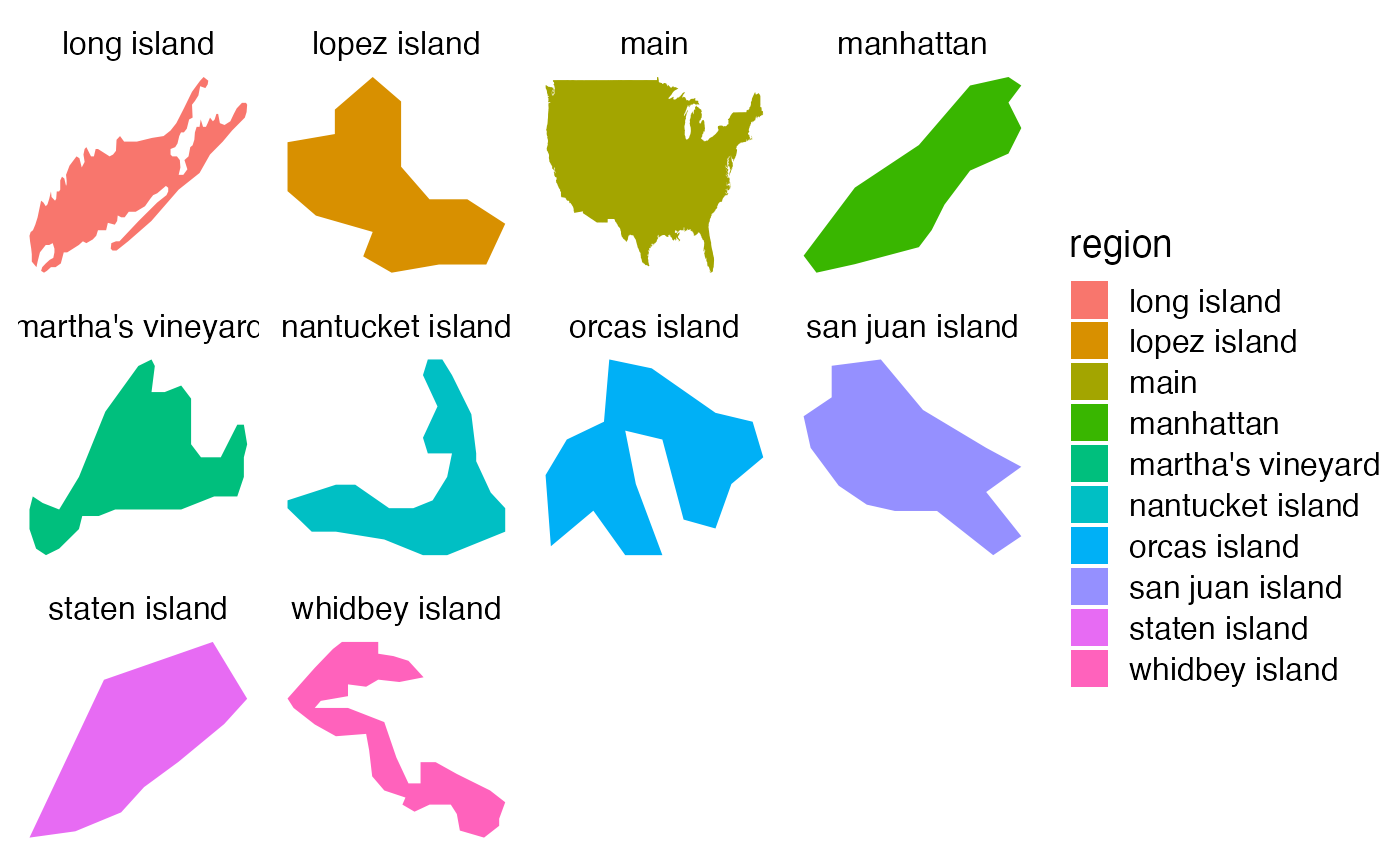The theme created by this function is useful for plotting maps with cowplot default sizing.

theme_map(
font_size = 14,
font_family = "",
line_size = 0.5,
rel_small = 12/14,
rel_tiny = 11/14,
rel_large = 16/14
)

## Arguments

font_size Overall font size. Default is 14. Base font family. Line size for axis lines. Relative size of small text (e.g., axis tick labels) Relative size of tiny text (e.g., caption) Relative size of large text (e.g., title)

The theme.

## Examples

library(ggplot2)
library(maps)

usa_data = map_data("usa")
ggplot(usa_data, aes(long, lat, fill = region)) +
geom_polygon() + theme_map()ggplot(usa_data, aes(long, lat, fill = region)) +
facet_wrap(~region, scales = "free") +
geom_polygon() + theme_map()